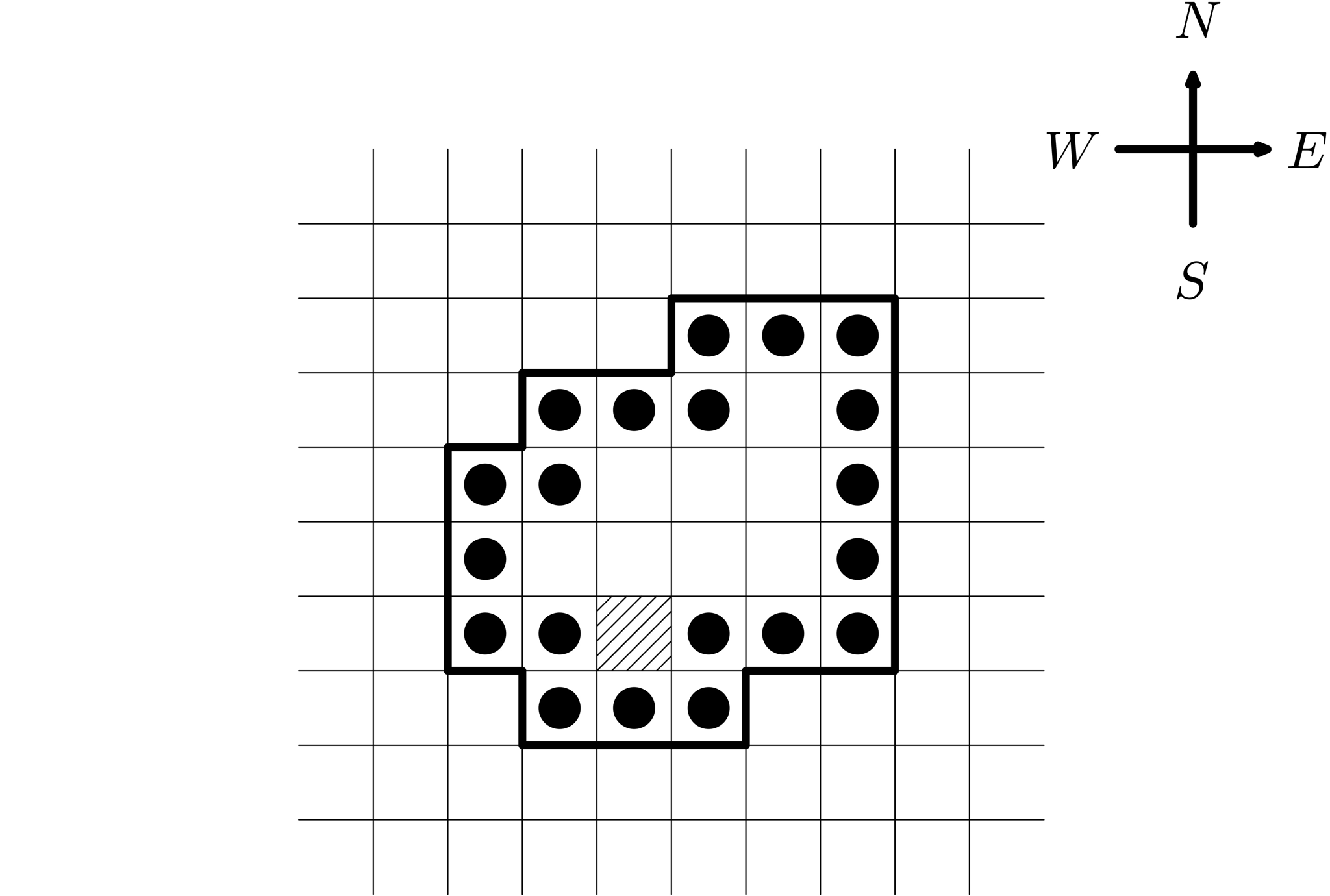시간 제한 메모리 제한 제출 정답 맞은 사람 정답 비율
2 초 256 MB 0 0 0 0.000%

## 문제

This is an interactive problem.

Consider an infinite square grid on the plane. Let us select a region on the grid: a set of grid squares. We define a regional path between squares $a$ and $b$ as a sequence of squares $c_1, c_2, \ldots, c_k$ where $c_1 = a$, $c_k = b$, all the squares belong to the region, and for all $i$ from $1$ to $k - 1$, squares $c_i$ and $c_{i + 1}$ share a common edge. The length of such path is $k$.

We say that a grid region is convex if for every pair of squares $(x_1, y_1)$ and $(x_2, y_2)$ which belong to the region, there is a regional path of length $|x_1 - x_2| + |y_1 - y_2|$ between them.

The jury selects a convex region and one special square in it. Let us call this square the central square.

Then the jury expands the region as follows. We take all the squares that do not belong to the region yet but have a common corner with a square from the region. We add all such squares to the region, and call the added squares the border of the region.

It is guaranteed that the region with the border can be fully covered by a $41 \times 41$ square aligned with the grid.

You have to find the area of the region using the following queries. Each query is represented by a program consisting of the commands "n", "s", "w", "e", indicating movement to the north, south, west, and east, respectively.

Before starting the program, the jury places one token on every border square. Then, each token moves in accordance with the specified program. If the next command moves a token outside of the region, this command is ignored for that token. For each token, the jury counts the number of times it moved to the central square. The result of the query is the sum of these values for each token.

## 프로토콜

The participant program must interact with the jury program  by printing commands in one of the following formats:

• "? $s$'', where $s$ should be a non-empty string containing no more than $250$ characters "n", "s", "w", and "e", denoting the program. For this query, the jury program will output a single integer: the sum over all the tokens of the number of times which the respective token moved to the central square.
• "! $\mathit{ans}$", where $\mathit{ans}$ is the integer area of the region. After printing this command, the program should terminate immediately.

Your solution can make at most 1500 queries.

## 예제 입력 1

1
1
8
4
3


## 예제 출력 1

? e
? w
? ssssswww
? sssssee
? wewsss
! 29


## 힌트

In each test for this problem, the region and the central square are fixed in advance and do not change.

The example corresponds to the following image:## 채점

• 예제는 채점하지 않는다.